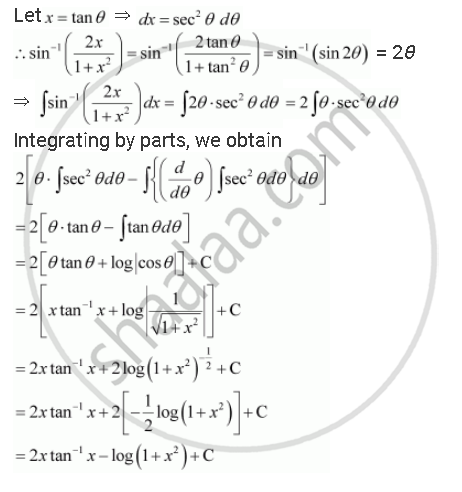Share

# Integrate the Functions Sin^(-1) ((2x)/(1+X^2)) - CBSE (Commerce) Class 12 - Mathematics

ConceptMethods of Integration - Integration by Parts

#### Question

Integrate the functions sin^(-1) ((2x)/(1+x^2))

#### SolutionIs there an error in this question or solution?

#### APPEARS IN

Solution Integrate the Functions Sin^(-1) ((2x)/(1+X^2)) Concept: Methods of Integration - Integration by Parts.
S